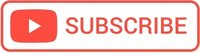# PHP Control Structures and Loops# PHP Conditional Statements: if else, switch

## If statement

Execute the code if the condition is true

## If…else statement

$num = 25; if($num % 2 ==0){
echo "the number $num is even"; }else{ echo "the number$num is odd";
}
//the number 25 is odd


Whitespace doesn’t matter. That’s the nature of a C-based programming language.

## Switch statement

Execute different code depending on a condition.
The switch statement is similar to a series of IF statements

switch ($var) { case value1: code will be executed if$var matches the value1;
break;
case value2:
code will be executed if $var matches the value2; break; case value3: case value4: code will be executed if$var matches the value3 or the value4;
break;
...
default:
do this if nothing else matches;
}


If the value pass to the switch statement matches, the code that follows the case is executed.

	$degrees = "23"; switch($degrees){
case ($degrees < 10): echo "it's very cold outside"; break; case ((10 <=$degrees) && ($degrees < 20)): echo "it's cold outside"; break; case ((20 <=$degrees) && ($degrees < 30)): echo "it's nice outside"; break; case ((30 <=$degrees) && ($degrees < 40)): echo "it's hot outside"; break; case ((40 <=$degrees) && ($degrees < 45)): echo "it's very hot outside"; break; default: echo "I do not know what it's like outside"; } //The result: it's nice outside  Be careful! if you forget to put the break, the code after “case” it will run even if will not match the value!!! $degrees = "23";
switch($degrees){ case ($degrees < 10):
echo "it's very cold outside ";
break;
case ((10 <= $degrees) && ($degrees < 20)):
echo "it's cold outside ";
break;
case ((20 <= $degrees) && ($degrees < 30)):
echo "it's nice outside ";
case ((30 <= $degrees) && ($degrees < 40)):
echo "it's hot outside ";
break;
case ((40 <= $degrees) && ($degrees < 45)):
echo "it's very hot outside ";
break;
default:
echo "I do not know what it's like outside";
} //The result: it's nice outside it's hot outside


## PHP while loop

A while loop start to test condition before first iteration.

$x = 1; while($x < 10) {
echo "$x,";$x++;
}
//Result 1,2,3,4,5,6,7,8,9,


## PHP Do-while loop

A do-while loop test condition at the bottom after completing the first iteration.

$x = 1; do{ echo "$x,";
$x++; }while($x < 10);
//Result 1,2,3,4,5,6,7,8,9,


## PHP for Loop

The for loop is used when you know how many times the script should run.

for (init counter; test counter; increment counter) {
code to be executed for each iteration;
}

for($x =1;$x<10; $x++){ echo "$x,";
}


## Breaking out of a Loop

The break statements ends the current loop and jumps to the statement immediately following the loop.

for($x =1;$x<10; $x++){ echo "$x,";
if($x ==5)break; } //1,2,3,4,5,  ## Continue The continue statements ends the current iteration, jums to the top of the loop and starts the next iteration. for($x =1; $x<10;$x++){
if($x ==5)continue; echo "$x,";
}
//1,2,3,4,6,7,8,9,


## PHP foreach Loop

The easiest way to iterate through each element of an array is to use foreach() function.

foreach loop works only on arrays, and is used to loop through each key/value pair in an array.

$capital = ['Paris','Berlin', 'London']; foreach($capital as $city){ echo "$city, ";
}
//Paris, Berlin, London,


#### Hello there!

I hope you find this post useful!

I'm Mihai, a programmer and online marketing specialist, very passionate about everything that means online marketing, focused on eCommerce.

If you have a collaboration proposal or need helps with your projects feel free to contact me. I will always be glad to help you!